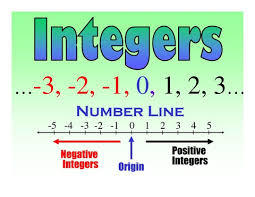Integers
3 years ago
afergurs
Save
Edit
Host a game
Live GameLive
Homework
Solo Practice
Practice18 QuestionsShow answers
• Question 1
120 seconds
Q. -15 - 3
12
-12
-18
18
• Question 2
30 seconds
Q.

-2 + 4

-2

2

0

-4

• Question 3
120 seconds
Q. 3 + -7
10
4
-10
-4
• Question 4
120 seconds
Q. -9 + (-5)
-14
14
-4
• Question 5
30 seconds
Q.

10 + (-4) = ?

-6

6

14

-14

• Question 6
120 seconds
Q. -7 - 2
-9
9
5
-5
• Question 7
120 seconds
Q. -8 - (-4) =
12
-12
4
-4
• Question 8
120 seconds
Q. 5-19
14
24
-14
-24
• Question 9
120 seconds
Q. -2 - (-10) = ?
-12
12
8
-8
• Question 10
120 seconds
Q. 0 - 5
-5
5
0
10
• Question 11
120 seconds
Q. The temperature changed from 3 degrees below zero at midnight to 7 degrees below zero at 2 a.m. How much did the temperature decrease?
5 degrees
4 degrees
10 degrees
it stayed the same
• Question 12
120 seconds
Q. 0 + (-100)
100
-100
0
200
• Question 13
120 seconds
Q. The high temperature Monday was 4 degrees. The low temperature was -7 degrees. What is the difference between the high and low temperatures?
3 degrees
12 degrees
11 degrees
10 degrees
• Question 14
120 seconds
Q. 80 + (-8)
88
-88
72
-72
• Question 15
120 seconds
Q. -19 - (-10)
29
-29
-9
9
• Question 16
120 seconds
Q. 35 - (-35)
0
-70
70
1
• Question 17
120 seconds
Q. The temperature at 6:00 was 9 degrees below zero.  The temperature dropped to 11 degrees below zero at 8:00. What is the difference between temperatures?
18 degrees
20 degrees
2 degrees
0 degrees
• Question 18
120 seconds
Q. -90 - 0